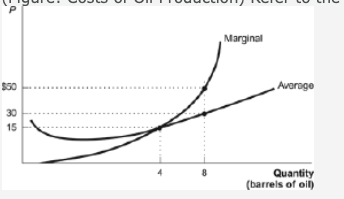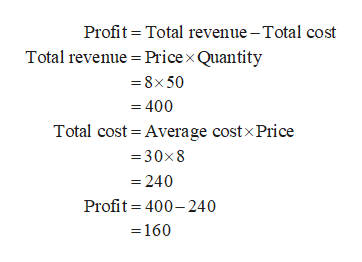Question
13 views
(Figure: Costs of Oil Production) Refer to the figure. Assuming that price equals marginal cost, the profit of producing eight barrels of oil is:

 \$160. \$400 It cannot be determined from the information given. \$240.help_outlineImage TranscriptioncloseMarginal Avorage \$50 30 15 Quantity (barrels of oil) fullscreen
check_circle

Step 1

Total profit refers to the remaining total revenue of a firm after deducting all the costs.

Step 2

In the given information, the price of 8 barrels of oil is \$50, which is equal to the marginal costs of the firm and the average...help_outlineImage TranscriptioncloseProfit = Total revenue – Total cost Total revenue = Pricex Quantity = 8x 50 = 400 Total cost = Average costx Price = 30x8 = 240 Profit = 400-240 =160 fullscreen

### Want to see the full answer?

See Solution

#### Want to see this answer and more?

Solutions are written by subject experts who are available 24/7. Questions are typically answered within 1 hour.*

See Solution
*Response times may vary by subject and question.
Tagged in